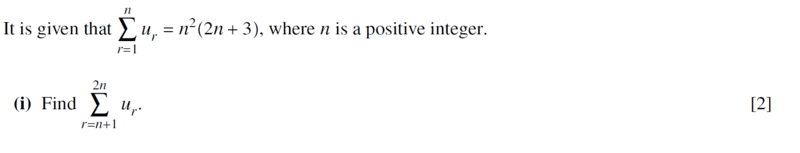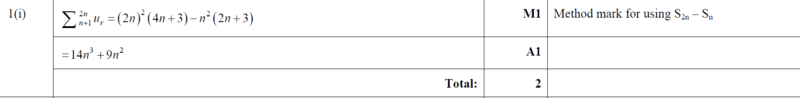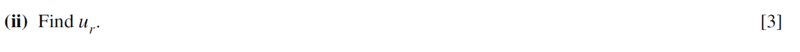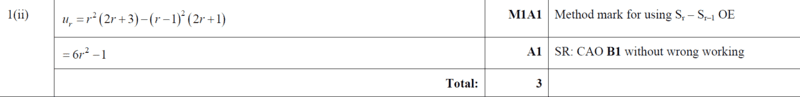# Find the sum of the series ##\sum_{r=n+1}^{2n} u_r##

Gold Member
Homework Statement:
See attached
Relevant Equations:
Sum of series
Find question and solution herePart (i) is clear to me as they made use of,

$$\sum_{r=n+1}^{2n} u_r=\sum_{r=1}^{2n} u_r-\sum_{r=1}^{n} u_r$$
to later give us the required working to solution...
...
##4n^2(4n+3)-n^2(2n+3)=16n^3+12n^2-2n^3-3n^2=14n^3+9n^2## as indicated.

My question is on the second part,I can see that they still made use of
let ##r=2n, ⇒1n=r-1## is that correct? giving us

##4n^2(4n+3)-n^2(2n+3)=r^2(2⋅r+3)-(r-1)^2(2(r-1)+3)##

##4n^2(4n+3)-n^2(2n+3)=r^2(2r+3)-(r-1)^2(2r+1)=6r^2-1##

I need more insight on the highlighted part. Thanks

Mentor
2022 Award
Homework Statement:: See attached
Relevant Equations:: Sum of series

Find question and solution here

View attachment 302024

View attachment 302025

Part (i) is clear to me as they made use of,

$$\sum_{r=n+1}^{2n} u_r=\sum_{r=1}^{2n} u_r-\sum_{r=1}^{n} u_r$$
to later give us the required working to solution...
...
##4n^2(4n+3)-n^2(2n+3)=16n^3+12n^2-2n^3-3n^2=14n^3+9n^2## as indicated.

My question is on the second part,

View attachment 302026

View attachment 302027

I can see that they still made use of
let ##r=2n, ⇒1n=r-1## is that correct? giving us

##4n^2(4n+3)-n^2(2n+3)=r^2(2⋅r+3)-(r-1)^2(2(r-1)+3)##

##4n^2(4n+3)-n^2(2n+3)=r^2(2r+3)-(r-1)^2(2r+1)=6r^2-1##

I need more insight on the highlighted part. Thanks
Why don't you use ##u_r=\sum_{k=1}^r u_k - \sum_{k=1}^{r-1} u_k##?

Gold Member
Why don't you use ##u_r=\sum_{k=1}^r u_k - \sum_{k=1}^{r-1} u_k##?
@fresh_42 let me check it out...

Gold Member
Why don't you use ##u_r=\sum_{k=1}^r u_k - \sum_{k=1}^{r-1} u_k##?
...but isn't that what i used? let me copy paste it here,

let ##r=2n, ⇒1n=r-1## is that correct? giving us ...

Mentor
2022 Award
...but isn't that what i used? let me copy paste it here,

let ##r=2n, ⇒1n=r-1## is that correct? giving us ...
I don't know. I gave up reading when I saw let ##r=2n##. How could this help to find ##u_r##. The reference to ##2n## and ##n-1## is misleading, to say the least.

•chwala
Mentor
2022 Award
Your solution is correct. Now that I did the algebra I ended up with the same result.

Gold Member
Your solution is correct. Now that I did the algebra I ended up with the same result.

Mentor
2022 Award
But what was your question? Which kind of insight were you looking for?
I would write it down as
\begin{align*}
u_r&=\sum_{k=1}^r u_k-\sum_{k=1}^{r-1} u_k\\
&=r^2(2r+3)-(r-1)^2(2(r-1)+3)\\
&\ldots \\
&=6r^2-1
\end{align*}

Gold Member
But what was your question? Which kind of insight were you looking for?
I would write it down as
\begin{align*}
u_r&=\sum_{k=1}^r u_k-\sum_{k=1}^{r-1} u_k\\
&=r^2(2r+3)-(r-1)^2(2(r-1)+3)\\
&\ldots \\
&=6r^2-1
\end{align*}
The change of variable from ##2n## to ##r## that was the part i needed more insight...could we as well have ##r+1## and ##r##? or ##r+2## and ##r+1##? That is my question...

Mentor
2022 Award
The change of variable from ##2n## to ##r## that was the part i needed more insight...

You can also check the result with the known formulas
##\sum_{r=1}^n r^2 = \dfrac{1}{6} n (n + 1) (2 n + 1)## and ##\sum_{r=1}^n 1= n## and see whether ##\sum_{r=1}^n u_r=\sum_{r=1}^n (6n^2-1) ## yields the original formula in post #1.

Gold Member

You can also check the result with the know formulas
##\sum_{r=1}^n r^2 = \dfrac{1}{6} n (n + 1) (2 n + 1)## and ##\sum_{r=1}^n 1= n## and see whether ##\sum_{r=1}^n u_r=\sum_{r=1}^n (6n^2-1) ## yields the original formula in post #1.
Post ##8## is clear to me...

Gold Member
•